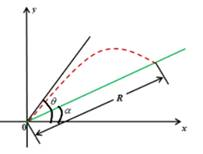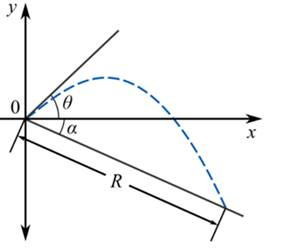# The range of the projectile is given by R ( θ ) = 2 v 2 cos θ sin ( θ − α ) g cos 2 θ .### Single Variable Calculus: Concepts...

4th Edition
James Stewart
Publisher: Cengage Learning
ISBN: 9781337687805### Single Variable Calculus: Concepts...

4th Edition
James Stewart
Publisher: Cengage Learning
ISBN: 9781337687805

#### Solutions

Chapter 4, Problem 64RE

(a)

To determine

## To show: The range of the projectile is given by R(θ)=2v2cosθ sin(θ−α)gcos2θ.

Expert Solution

### Explanation of Solution

Given information:

Parabola is y=(tanθ)xg2v2cos2θx2 0θπ2 .

Calculation:Consider that y=(tanθ)xg2v2cos2θx2 0θπ2

From the figure tanα=yx and y=xtanα

Thus,

xtanα=(tanθ)xg2v2cos2θx2tanα=(tanθ)g2v2cos2θxx=(tanθtanα)2v2cos2θg

Next rewrite tanθ=sinθcosθ and tanα=sinαcosα

x=(sinθcosθsinαcosα)2v2cos2θgx=(sinθcosαcosθsinαcosθcosα)2v2cos2θgx=(sin(θα)cosα)2v2cosθg

From the figure cosα=xRx=Rcosα

So,

Rcosα=(sin(θα)cosα)2v2cosθgR=(sin(θα)cos2α)2v2cosθgR=2v2cosθsin(θα)gcos2α

Hence R=2v2cosθsin(θα)gcos2α proved.

(b)

To determine

### To find: The θ so that R is maximum.

Expert Solution

The absolute maximum of R occurs when θ=π4+α2 .

### Explanation of Solution

Given information:

The given information is

Parabola y=(tanθ)xg2v2cos2θx2 0θπ2 .

Calculation:

To maximize R , find the derivative of R with respect to

Use the identity cos(A+B)=cosAcosBsinASinB

R'=2v2gcos2α[cosθcos(θα)sinθsin(θα)]=2v2gcos2α[cos(θ+(θα))]=2v2gcos2α[cos(2θα)]

The equation R'=0 is satisfied when cos(2θα)=0 that is, when

θ=π2+α2=π4+α2

Thus R'=0 when θ=π4+α2 . Observe that R'<0 when θ>π4+α2 and R'>0 when θ<π4+α2 ,so by the first derivative Test.

Therefore, the absolute maximum of R occurs when θ=π4+α2 .

(c)

To determine

### To find: The range R and angle at which projectile should be fired to maximize R.

Expert Solution

The absolute maximum of R occurs when θ=π4α2 .

### Explanation of Solution

Given information:

The given information is Parabola y=(tanθ)xg2v2cos2θx2 0θπ2 .

Calculation:

Draw a diagram of projectile.From the figure tanα=yx and y=xtanα

Thus,

xtanα=(tanθ)xg2v2cos2θx2tanα=(tanθ)g2v2cos2θxx=(tanθ+tanα)2v2cos2θg

Next rewrite tanθ=sinθcosθ and tanα=sinαcosα

x=(sinθcosθ+sinαcosα)2v2cos2θgx=(sinθcosα+cosθsinαcosθcosα)2v2cos2θgx=(sin(θ+α)cosα)2v2cosθg

From the figure cosα=xRx=Rcosα

So,

Rcosα=(sin(θ+α)cosα)2v2cosθgR=(sin(θ+α)cos2α)2v2cosθgR=2v2cosθsin(θ+α)gcos2α

To maximize, find the derivative of R with respect to

R'=2v2gcos2α[cosθcos(θ+α)sinθsin(θ+α)]=2v2gcos2α[cos(θ+(θ+α))]=2v2gcos2α[cos(2θ+α)]

The equation R'=0 is satisfied when cos(2θ+α)=0 that is, when

θ=π2α2=π4α2

Thus, the absolute maximum of R occurs when θ=π4α2 .

### Have a homework question?

Subscribe to bartleby learn! Ask subject matter experts 30 homework questions each month. Plus, you’ll have access to millions of step-by-step textbook answers!